Name:    Quiz 2.1-2.4

Multiple Choice
Identify the choice that best completes the statement or answers the question.

Estimate the product or quotient.

1.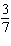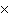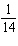a. 0 c.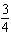b. 1 d.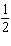2.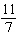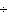3
 a.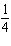c. 0 b. 1 d.3.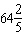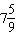a. 5 c. 12 b. 8 d. 9

Use compatible numbers to estimate the product or quotient.

4.

61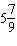a. 7 c. 10 b. 8 d. 6

5.

8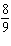a.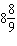c.b.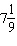d.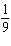6.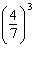a.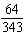c.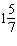b.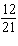d.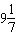Use the Distributive Property to find the product.

7.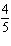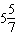a.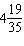c.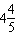b.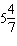d.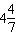8.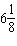a.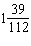c.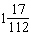b.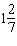d.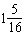9.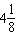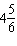5
 a.c.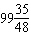b.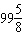d. 80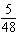Evaluate the expression when x =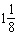, y =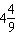, and z =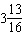.

10.

xz
 a.c.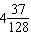b.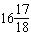d.11.

x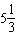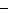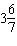a.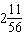c.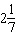b.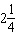d.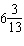Numeric Response

Estimate the product or quotient.

1.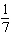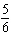2.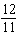2

1.

Find a fraction that when multiplied byis less than.

2.

You are going to make a cement patio with a garden in the space shown at the left. The dimensions of the garden are shown at the right.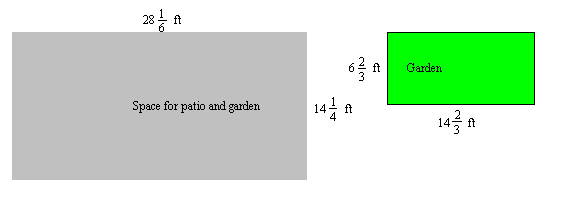a.   Draw a diagram that shows your layout of the garden and patio.
b.   Describe two methods for finding the area of the cement patio.
c.   Find the area of the cement patio. Which method did you use, and why?